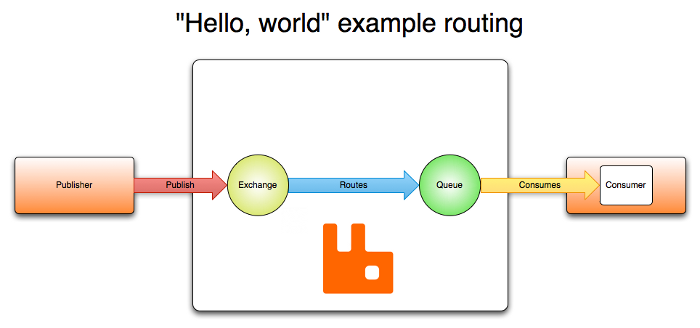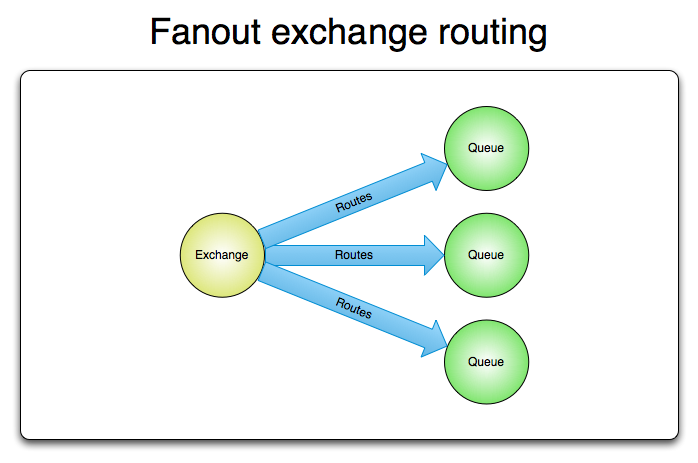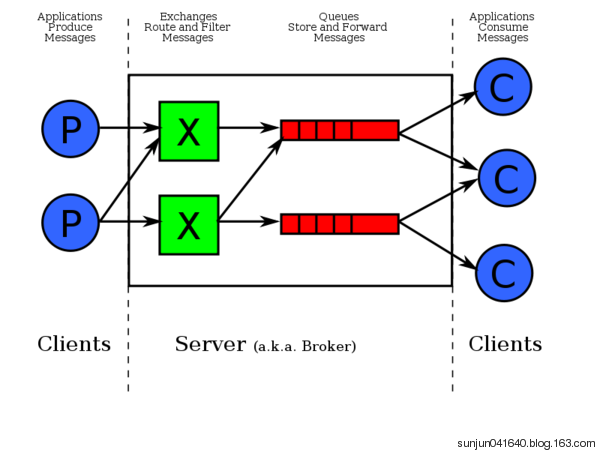# Rabbit MQ

2019/01/27 21:26

## AMQP简介

介绍rabbitMQ之前。先介绍一下AMQP协议，因为rabbitMQ是基于AMQP协议实现的一个服务程序。（目前为止应该也是唯一实现了AMQP协议的服务）

### AMQP定义

AMQP（高级消息队列协议）是一个网络协议。它支持符合要求的客户端应用（application）和消息中间件代理（messaging middleware brockers）之间进行通信。

### AMQP 0-9-1 模型简介

AMQP 0-9-1的工作过程如下图：消息（message）被发布者（publisher）发送给交换机（exchange），交换机常常被比喻成邮局或者邮箱。然后交换机将收到的消息根据路由规则分发给绑定的队列（queue）。最后AMQP代理会将消息投递给订阅了此队列的消费者，或者消费者按照需求自行获取。### AMQP是一个可编的程协议

AMQP 0-9-1是一个可编程协议，某种意义上说AMQP的实体和路由规则是由应用本身定义的，而不是由消息代理定义。包括像声明队列和交换机，定义他们之间的绑定，订阅队列等等关于协议本身的操作。

## 交换机和交换机类型

Name（交换机类型） Default pre-declared names（预声明的默认名称）
Direct exchange（直连交换机） (Empty string) and amq.direct
Fanout exchange（扇型交换机） amq.fanout
Topic exchange（主题交换机） amq.topic

除交换机类型外，在声明交换机时还可以附带许多其他的属性，其中最重要的几个分别是：

• Name （交换机的名字）
• Type （交换机的种类）
• Passive （被动模式，布尔值）
• Durable （消息代理重启后，交换机是否还存在）
• Auto_delete （当所有与之绑定的消息队列都完成了对此交换机的使用后，删掉它）
• Arguments（依赖代理本身）

### 直连交换机

• 将一个队列绑定到某个交换机上，同时赋予该绑定一个路由键（routing key）
• 当一个携带着路由键为`R`的消息被发送给直连交换机时，交换机会把它路由给绑定值同样为`R`的队列。### 扇型交换机

• 大规模多用户在线（MMO）游戏可以使用它来处理排行榜更新等全局事件
• 体育新闻网站可以用它来近乎实时地将比分更新分发给移动客户端
• 分发系统使用它来广播各种状态和配置更新
• 在群聊的时候，它被用来分发消息给参与群聊的用户。（AMQP没有内置presence的概念，因此XMPP可能会是个更好的选择）### 主题交换机

• 分发有关于特定地理位置的数据，例如销售点
• 由多个工作者（workers）完成的后台任务，每个工作者负责处理某些特定的任务
• 股票价格更新（以及其他类型的金融数据更新）
• 涉及到分类或者标签的新闻更新（例如，针对特定的运动项目或者队伍）
• 云端的不同种类服务的协调
• 分布式架构/基于系统的软件封装，其中每个构建者仅能处理一个特定的架构或者系统。

## 队列

AMQP中的队列（queue）跟其他消息队列或任务队列中的队列是很相似的：它们存储着即将被应用消费掉的消息。队列跟交换机共享某些属性，但是队列也有一些另外的属性。

• Name
• Durable（消息代理重启后，队列依旧存在）
• Exclusive（只被一个连接（connection）使用，而且当连接关闭后队列即被删除）
• Auto_delete（当最后一个消费者退订后即被删除）
• Arguments（一些消息代理用他来完成类似与TTL的某些额外功能）

## 绑定

• 队列（queue）是我们想要去的位于纽约的目的地
• 交换机（exchange）是JFK机场
• 绑定（binding）就是JFK机场到目的地的路线。能够到达目的地的路线可以是一条或者多条

## 消费者

• 将消息投递给应用 ("push API")
• 应用根据需要主动获取消息 ("pull API")

### 消息确认

• 当消息代理（broker）将消息发送给应用后立即删除。（使用AMQP方法：basic.deliver或basic.get-ok）
• 待应用（application）发送一个确认回执（acknowledgement）后再删除消息。（使用AMQP方法：basic.ack）

## 消息属性和有效载荷（消息主体）

AMQP模型中的消息（Message）对象是带有属性（Attributes）的。有些属性及其常见，以至于AMQP 0-9-1 明确的定义了它们，并且应用开发者们无需费心思思考这些属性名字所代表的具体含义。例如：

• Content type（内容类型）
• Content encoding（内容编码）
• Routing key（路由键）
• Delivery mode (persistent or not)
投递模式（持久化 或 非持久化）
• Message priority（消息优先权）
• Message publishing timestamp（消息发布的时间戳）
• Expiration period（消息有效期）
• Publisher application id（发布应用的ID）

AMQP的消息除属性外，也含有一个有效载荷 - Payload（消息实际携带的数据），它被AMQP代理当作不透明的字节数组来对待。消息代理不会检查或者修改有效载荷。消息可以只包含属性而不携带有效载荷。它通常会使用类似JSON这种序列化的格式数据，为了节省，协议缓冲器和MessagePack将结构化数据序列化，以便以消息的有效载荷的形式发布。AMQP及其同行者们通常使用"content-type" 和 "content-encoding" 这两个字段来与消息沟通进行有效载荷的辨识工作，但这仅仅是基于约定而已。

## AMQP 0-9-1 方法

AMQP 0-9-1由许多方法（methods）构成。方法即是操作，这跟面向对象编程中的方法没半毛钱关系。AMQP的方法被分组在类（class）中。这里的类仅仅是对AMQP方法的逻辑分组而已。在 AMQP 0-9-1参考中有对AMQP方法的详细介绍。

• exchange.declare
• exchange.declare-ok
• exchange.delete
• exchange.delete-ok

（请注意，RabbitMQ网站参考中包含了特用于RabbitMQ的交换机类的扩展，这里我们不对其进行讨论）exchange.declare-ok方法除了通道号之外没有携带任何其他参数（通道-channel 会在本指南稍后章节进行介绍）。

AMQP队列类的配对方法 - queue.declare方法 和 queue.declare-ok有着与其他配对方法非常相似的一系列事件：不是所有的AMQP方法都有与其配对的“另一半”。许多（basic.publish是最被广泛使用的）都没有相对应的“响应”方法，另外一些（如basic.get）有着一种以上与之对应的“响应”方法。

## 连接

AMQP连接通常是长连接。AMQP是一个使用TCP提供可靠投递的应用层协议。AMQP使用认证机制并且提供TLS（SSL）保护。当一个应用不再需要连接到AMQP代理的时候，需要优雅的释放掉AMQP连接，而不是直接将TCP连接关闭。

## AMQP是可扩展的

AMQP 0-9-1 拥有多个扩展点：

• 定制化交换机类型 可以让开发者们实现一些开箱即用的交换机类型尚未很好覆盖的路由方案。例如 geodata-based routing。
• 交换机和队列的声明中可以包含一些消息代理能够用到的额外属性。例如RabbitMQ中的per-queue message TTL即是使用该方式实现。
• 特定消息代理的协议扩展。例如RabbitMQ所实现的扩展。
• 新的 AMQP 0-9-1 方法类可被引入。
• 消息代理可以被其他的插件扩展，例如RabbitMQ的管理前端 和 已经被插件化的HTTP API。

## AMQP 0-9-1 客户端生态系统

AMQP 0-9-1 拥有众多的适用于各种流行语言和框架的客户端。其中一部分严格遵循AMQP规范，提供AMQP方法的实现。另一部分提供了额外的技术，方便使用的方法和抽象。有些客户端是异步的（非阻塞的），有些是同步的（阻塞的），有些将这两者同时实现。有些客户端支持“供应商的特定扩展”（例如RabbitMQ的特定扩展）。

## RabbitMQ

### 安装

• 使用`sudo apt-get install rabbitmq-server`安装rabbitmq，之后开启rabbitmq的web管理页面
• 使用`sudo /usr/sbin/rabbitmq-plugins enable rabbitmq_management`开启rabbitmq的web管理页面插件
• 使用`sudo /etc/init.d/rabbitmq-server start`启动rabbitmq服务
• rabbitmq的连接端口默认是5672端口，可以使用`sudo netstat -ntlp`查看，如果开启了web管理页面的话，应该能看到5672,15672,25672几个端口被打开

### 实现最简单的队列通信

`# 启动rabbitMQ服务端<br>sudo /etc.init.d/rabbitmq-server start<br># 创建一个用户`
`sudo rabbitmqctl add_user gyc ` `123123`
`# 设置用户为administrator角色`
`dudo rabbitmqctl set_user_tags gyc administrator`
`# 设置权限`
`sudo rabbitmqctl set_permissions ` `-` `p ` `"/"`  `gyc ` `'.'` `'.'` `'.'`
`# 然后重启rabbiMQ服务`
`sudo ` `/` `etc` `/` `init.d` `/` `rabbitmq` `-` `server restart`

`# 然后可以使用刚才的用户远程连接rabbitmq server了。`### 应用

python连接rabbitmq需要安装一个包，pika，安装命令:`pip3 install pika`

rabbit_direct_publish.py

 1 2 3 4 5 6 7 8 9 10 11 12 13 14 15 16 17 18 19 20 21 22 23 24 25 26 27 28 `import`  `pika  ` `# 链接rabbitMQ` `import`  `sys`   `# 建立TCP链接` `credentials ` `=`  `pika.PlainCredentials(` `"gyc"` `, ` `"123123"` `)` `connection ` `=`  `pika.BlockingConnection(pika.ConnectionParameters(host` `=` `"192.168.14.63"` `, credentials` `=` `credentials))`   `# 建立一个通道` `channel ` `=`  `connection.channel()`   `# 声明交换机名称和类型` `channel.exchange_declare(exchange` `=` `"direct_logs"` `,` `                         ` `type` `=` `"direct"` `)`   `serverity ` `=`  `sys.argv[` `1` `] ` `if`  `len` `(sys.argv) > ` `1`  `else`  `"info"`   `# 获取routing_key`   `message ` `=`  `" "` `.join(sys.argv[` `2` `:]) ` `or`  `"info: Hello World"`   `# 获取消息内容`   `# RabbitMQ消息不能直接发送到队列,它需要经历一个交换的过程。` `channel.basic_publish(` `    ` `exchange` `=` `"direct_logs"` `,  ` `# 指定消息发送到的交换机名称` `    ` `routing_key` `=` `serverity,  ` `# 指定消息的routing_key` `    ` `body` `=` `message  ` `# 消息体` `)`   `print` `(` `"[x] Send 'Hello World!'"` `)`   `connection.close()`

server端

 1 2 3 4 5 6 7 8 9 10 11 12 13 14 15 16 17 18 19 20 21 22 23 24 25 26 27 28 29 30 31 32 33 34 35 36 37 38 39 40 41 42 `import`  `pika` `import`  `sys`   `print` `(sys.argv)`   `credentials ` `=`  `pika.PlainCredentials(` `"gyc"` `, ` `"123123"` `)` `connection ` `=`  `pika.BlockingConnection(pika.ConnectionParameters(host` `=` `"192.168.14.63"` `, credentials` `=` `credentials))`   `channel ` `=`  `connection.channel()`   `channel.exchange_declare(exchange` `=` `"direct_logs"` `,` `                         ` `type` `=` `"direct"` `)`   `result ` `=`  `channel.queue_declare(exclusive` `=` `True` `)  ` `# 不指定queue名字,rabbit会随机分配一个名字,exclusive=True会再使用此queue的消费者` `# 断开后,自动将queue删除` `queue_name ` `=`  `result.method.queue  ` `# 取rabbitmq-server返回的queue名称`   `serverities ` `=`  `sys.argv[` `1` `:]  ` `# 获取参数`   `if`  `not`  `serverities:  ` `# 如果没有参数会抛出异常,提示应该填写什么参数` `    ` `sys.stderr.write(` `"Usage: %s[info] [warning] [error]\n"`  `%`  `sys.argv[` `0` `])` `    ` `sys.exit(` `1` `)`   `for`  `serverity ` `in`  `serverities:  ` `# 将该队列通过不同的routing_key绑定到交换机direct_logs上` `    ` `channel.queue_bind(` `        ` `exchange` `=` `"direct_logs"` `,` `        ` `queue` `=` `queue_name,` `        ` `routing_key` `=` `serverity` `    ` `)`   `print` `(` `"[*] Waiting for logs. To exit press CTRL+C"` `)`     `def`  `callback(ch, method, properties, body):  ` `# 定义回调函数` `    ` `print` `(` `"[x] %r:%r"`  `%`  `(method.routing_key, body))`   `channel.basic_consume(` `    ` `callback,` `    ` `queue` `=` `queue_name,` `)`   `channel.start_consuming()  ` `# 启动侦听消息队列

# 注：sys参数需要到终端上执行该py文件填写`

rabbit_fanout_publish.py

 1 2 3 4 5 6 7 8 9 10 11 12 13 14 15 16 17 18 19 20 21 22 23 24 25 26 `import`  `pika`   `import`  `sys`   `credentials ` `=`  `pika.PlainCredentials(` `"gyc"` `, ` `"123123"` `)` `connection ` `=`  `pika.BlockingConnection(pika.ConnectionParameters(` `    ` `host` `=` `"192.168.14.63"` `,` `    ` `credentials` `=` `credentials` `))`   `channel ` `=`  `connection.channel()`   `channel.exchange_declare(exchange` `=` `"logs"` `,  ` `# 声明交换机logs 类型为fanout` `                         ` `type` `=` `"fanout"` `)`   `message ` `=`  `"` `".join(sys.argv[1:]) or "` `info: Hello World!"`   `channel.basic_publish(` `    ` `exchange` `=` `"logs"` `,  ` `# 指定消息发送到交换机的名称` `    ` `routing_key` `=` `"",  ` `# fanout类型为广播,所以不需要指定routing_key，所有连接到该交换机的消息队列都将能收到发过来消息` `    ` `body` `=` `message` `)`   `print` `(` `" [x] Send %r"`  `%`  `message)`   `connection.close()`

rabbit_fanout_consumer.py

 1 2 3 4 5 6 7 8 9 10 11 12 13 14 15 16 17 18 19 20 21 22 23 24 25 26 27 28 29 30 31 `import`  `pika`   `credentials ` `=`  `pika.PlainCredentials(` `"gyc"` `, ` `"3778627"` `)` `connection ` `=`  `pika.BlockingConnection(pika.ConnectionParameters(` `    ` `host` `=` `"192.168.14.63"` `,` `    ` `credentials` `=` `credentials` `))`   `channel ` `=`  `connection.channel()`   `channel.exchange_declare(exchange` `=` `"logs"` `, ` `type` `=` `"fanout"` `)`   `result ` `=`  `channel.queue_declare(exclusive` `=` `True` `)` `queue_name ` `=`  `result.method.queue`   `channel.queue_bind(exchange` `=` `"logs"` `,` `                   ` `queue` `=` `queue_name)`   `print` `(` `"[*] Waiting for logs. To exit press CTRL+C"` `)`     `def`  `callback(ch, method, properties, body):` `    ` `print` `(` `"[x] %r"`  `%`  `body)`   `channel.basic_consume(` `    ` `callback,` `    ` `queue` `=` `queue_name,` `    ` `no_ack` `=` `True` `)`   `channel.start_consuming()`

rabbit_topic_publish.py

 1 2 3 4 5 6 7 8 9 10 11 12 13 14 15 16 17 18 19 20 21 22 23 24 25 26 27 28 `import`  `pika`   `import`  `sys`   `credentials ` `=`  `pika.PlainCredentials(` `"gyc"` `, ` `"123123"` `)`   `connection ` `=`  `pika.BlockingConnection(pika.ConnectionParameters(` `    ` `host` `=` `"192.168.14.63"` `, credentials` `=` `credentials` `))`   `channel ` `=`  `connection.channel()`   `channel.exchange_declare(exchange` `=` `"topic_logs"` `,` `                         ` `type` `=` `"topic"` `)`   `routing_key ` `=`  `sys.argv[` `1` `] ` `if`  `len` `(sys.argv) > ` `1`  `else`  `"anonymous.info"`   `message ` `=`  `"` `".join(sys.argv[2:]) or "` `Hello World!"`   `channel.basic_publish(` `    ` `exchange` `=` `"topic_logs"` `,` `    ` `routing_key` `=` `routing_key,` `    ` `body` `=` `message` `)`   `print` `(` `" [x] Send %r:%r"`  `%`  `(routing_key, message))`   `connection.close()`

rabbit_topic_consumer.py

 1 2 3 4 5 6 7 8 9 10 11 12 13 14 15 16 17 18 19 20 21 22 23 24 25 26 27 28 29 30 31 32 33 34 35 36 37 38 `import`  `pika`   `import`  `sys`   `credentials ` `=`  `pika.PlainCredentials(` `"gyc"` `, ` `"3778627"` `)` `connection ` `=`  `pika.BlockingConnection(pika.ConnectionParameters(` `    ` `host` `=` `"192.168.14.63"` `, credentials` `=` `credentials` `))`   `channel ` `=`  `connection.channel()`   `channel.exchange_declare(exchange` `=` `"topic_logs"` `,` `                         ` `type` `=` `"topic"` `)`   `result ` `=`  `channel.queue_declare(exclusive` `=` `True` `)` `queue_name ` `=`  `result.method.queue`   `binding_keys ` `=`  `sys.argv[` `1` `:]`   `if`  `not`  `binding_keys:` `    ` `sys.stderr.write(` `"Usage: %s [binding_keys]\n"`  `%`  `sys.argv[` `0` `])` `    ` `sys.exit()`   `for`  `binding_key ` `in`  `binding_keys:` `    ` `channel.queue_bind(exchange` `=` `"topic_logs"` `,` `                       ` `queue` `=` `queue_name,` `                       ` `routing_key` `=` `binding_key)`   `print` `(` `"[*] Waiting for logs. To exit press CTRL+C"` `)`     `def`  `callback(ch, method, properties, body):` `    ` `print` `(` `"[x] %r:%r"`  `%`  `(method.routing_key, body))`   `channel.basic_consume(callback, queue` `=` `queue_name)`   `channel.start_consuming()` `# 注:binding_keys就是你要设置的消息的键，需要在publish侧的routing_key能匹配到`

rpc_client.py

 1 2 3 4 5 6 7 8 9 10 11 12 13 14 15 16 17 18 19 20 21 22 23 24 25 26 27 28 29 30 31 32 33 34 35 36 37 38 39 40 41 42 43 44 45 46 47 48 49 50 51 52 53 54 55 56 57 58 `import`  `pika`   `import`  `uuid`     `class`  `FibonacciRpcClient:` `    ` `def`  `__init__(` `self` `):` `        ` `self` `.credentials ` `=`  `pika.PlainCredentials(` `"gyc"` `, ` `"123123"` `)` `        ` `self` `.connection ` `=`  `pika.BlockingConnection(pika.ConnectionParameters(` `            ` `host` `=` `"192.168.14.63"` `, credentials` `=` `self` `.credentials` `        ` `))  ` `# 初始化, 建立TCP连接,前面加self是因为我们要在其他地方进行调用`   `        ` `self` `.channel ` `=`  `self` `.connection.channel()`   `        ` `result ` `=`  `self` `.channel.queue_declare(exclusive` `=` `True` `)` `        ` `self` `.callback_queue ` `=`  `result.method.queue`   `        ` `self` `.channel.basic_consume(` `self` `.on_response, no_ack` `=` `True` `, queue` `=` `self` `.callback_queue)  ` `# 绑定回调函数和消息队列`   `    ` `def`  `on_response(` `self` `, ch, method, props, body):  ` `# 定义回调函数` `        ` `if`  `self` `.corr_id ` `=` `=`  `props.correlation_id:` `            ` `self` `.response ` `=`  `body`   `    ` `def`  `call(` `self` `, n):` `        ` `self` `.response ` `=`  `None` `        ` `self` `.corr_id ` `=`  `str` `(uuid.uuid4())  ` `# 获取UUID, 随机唯一值,用来标识返回的数据是客户端所需要的` `        ` `self` `.channel.basic_publish(exchange` `=` `"",  ` `# 以routing_key为rpc_queue，在消息头中定义reply_to告诉server消息回给client生成的消息队列，并且消息的correlation_id为client自己生成的uuid，server回消息时也将会带上这个uuid` `                                   ` `routing_key` `=` `"rpc_queue"` `,` `                                   ` `properties` `=` `pika.BasicProperties(` `                                       ` `reply_to` `=` `self` `.callback_queue,` `                                       ` `correlation_id` `=` `self` `.corr_id,` `                                   ` `),  ` `# 告诉server端回消息的队列,返回的时候带上这个uuid` `                                   ` `body` `=` `str` `(n))  ` `# 消息体` `        ` `while`  `self` `.response ` `is`  `None` `:  ` `# 循环检测self.response是否有返回值` `            ` `self` `.connection.process_data_events()` `            ` `'''Will make sure that data events are processed. Dispatches timer and` `            ` `channel callbacks if not called from the scope of BlockingConnection or` `            ` `BlockingChannel callback. Your app can block on this method.`   `            ` `:param float time_limit:suggested upper bound on processing time in` `            ` `seconds. The actual blocking time depends on the granularity of the` `            ` `underlying ioloop. Zero means return as soon as possible. None means` `            ` `there is no limit on processing time and the function will block` `            ` `until I/O produces actionalable events. Defaults to 0 for backward` `            ` `compatibility. This parameter is NEW in pika 0.10.0.` `            ` `该方法可以传递参数time_limit=0，默认为0，即为不阻塞的检测channel是否有消息回来，如果` `            ` `有消息接收到，则执行回调函数，当time_limit不为0时，将每次检测阻塞time_limit秒。该方法` `            ` `在pika0.10.0中新加。` `            ` `'''` `        ` `return`  `int` `(` `self` `.response)`   `fibonacci_rpc ` `=`  `FibonacciRpcClient()`   `print` `(` `"[x] Requesting fib(30)"` `)`   `response ` `=`  `fibonacci_rpc.call(` `30` `)`   `print` `(` `"[.] Got %r"`  `%`  `response)`

rpc_server.py

`import`  `pika`

`credentials ` `=`  `pika.PlainCredentials(` `"gyc"` `, ` `"123123"` `)`

`connection ` `=`  `pika.BlockingConnection(pika.ConnectionParameters(`
`    ` `host` `=` `"192.168.14.63"` `, credentials` `=` `credentials`
`))`

`channel ` `=`  `connection.channel()`

`channel.queue_declare(queue` `=` `"rpc_queue"` `) ` `# 声明queue名称为rpc_queue`

`def`  `fib(n):`
`    ` `# 斐波那契计算函数`
`    ` `if`  `n ` `=` `=`  `0` `:`
`        ` `return`  `0`
`    ` `elif`  `n ` `=` `=`  `1` `:`
`        ` `return`  `1`
`    ` `else` `:`
`        ` `return`  `fib(n ` `-`  `1` `) ` `+`  `fib(n ` `-`  `2` `)`

`def`  `on_request(ch, method, props, body):`
`    ` `n ` `=`  `int` `(body)`

`    ` `print` `(` `"[.] fib(%s)"`  `%`  `n)`

`    ` `response ` `=`  `fib(n)`

`    ` `ch.basic_publish(exchange` `=` `"",`
`                     ` `routing_key` `=` `props.reply_to,`
`                     ` `properties` `=` `pika.BasicProperties(`
`                         ` `correlation_id` `=` `props.correlation_id`
`                     ` `),`
`                     ` `body` `=` `str` `(response))`

`    ` `ch.basic_ack(delivery_tag` `=` `method.delivery_tag)  ` `# 给rabbitmq_server发送ack确认消息`

`channel.basic_qos(prefetch_count` `=` `1` `)`

`channel.basic_consume(on_request, queue` `=` `"rpc_queue"` `)`

`print` `(` `"[x] Waiting RPC requests"` `)`

`channel.start_consuming()`

转自 https://www.cnblogs.com/sxzwj/p/6422870.html

0
0 收藏

### 作者的其它热门文章0 评论
0 收藏
0